# Visualising Solid Shapes Class 7 Notes: Chapter 15

## What are Solids in Maths?

Dimensions

• Dimension is a measurable length along a direction.
• Dimensions are length, breadth (or width) or height (or depth).
• A point is dimensionless.
• Based on the number of dimensions a figure has, there are one-dimensional, two-dimensional and three-dimensional figures.

## You Already Know These Shapes!!

1D and 2D figures

• One-dimensional figures do not enclose any area and have only one direction.
Example: A ray or a line segment.
• Two-dimensional figures have an area associated with them.
They have length and breadth or width.
They are usually plane figures, like squares, rectangles and circles.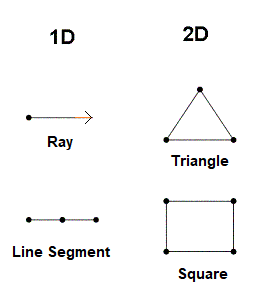To know more about 2D Shapes, visit here.
Solid Shapes

• Solid shapes have length, breadth or width and depth or height.
• They are called 3D or three-dimensional shapes.
• Example: Cuboids, Cylinders, Spheres and Pyramids.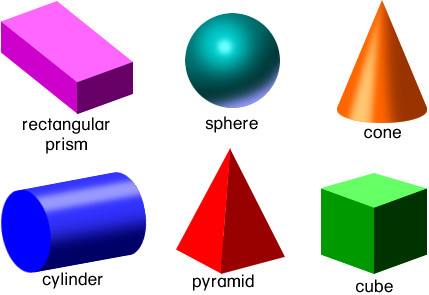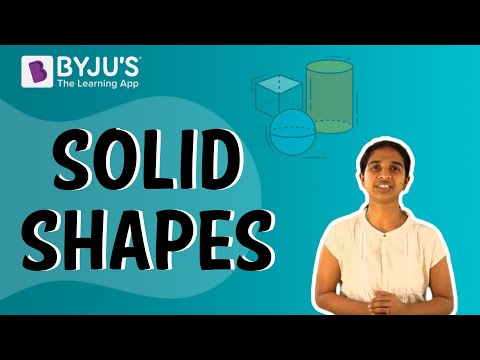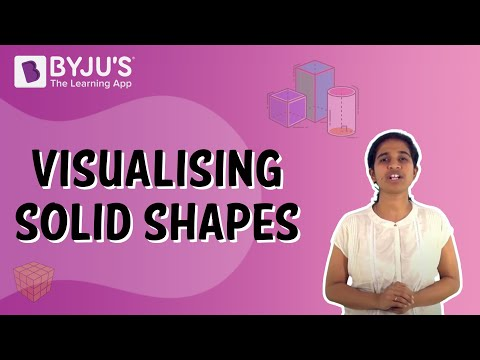## Perspectives of a Solid Shape

Visualising solid shapes

• Solid shapes can be visualised in different ways.
• Solid shapes can be formed by placing some solid shapes side by side to form a new solid shape.
• Example: (i) A cylinder is formed by stacking up circles vertically.
(ii) Cubes can be formed by placing cubes side by side.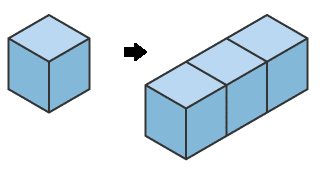To know more about Visualising Solid Shapes, visit here.
Visualising solid objects by viewing the smallest unit

• A solid can be visualised by breaking it down to the smallest unit.
• For example, a cube of dimensions (3cm x 3cm x 3cm) can be made up of 27 small cubes of dimensions (1cm x 1cm x 1cm).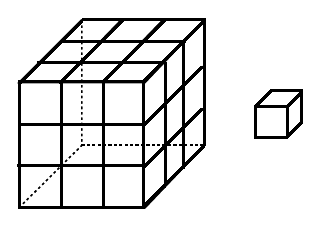Viewing sections of a solid by slicing or cutting

• If you cut or slice a solid we get to view the cross-section.
Example: If a loaf of bread is cut vertically, then the crosssection is almost a square. But if it is cut or sliced horizontally, the cross-section is a rectangle.
• Similarly, other solid shapes can be sliced in order to find their 2D cross-sectional shapes.
Example: A cucumber cut vertically gives a circle.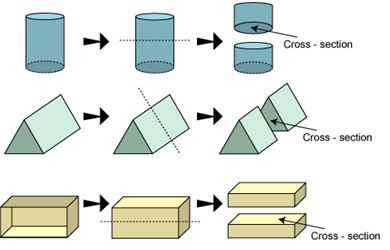Viewing sections of a solid by using shadows

• Shadows can be used to view 3D objects as 2D shapes.
• Example: The shadows of a cylinder are shown below.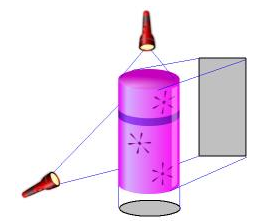Different views of a Solid

• A solid can be visualised by looking at it from different angles, namely: (i) Front view (ii) Top view (iii) Side view.
• Example: The different views of a figure are shown below.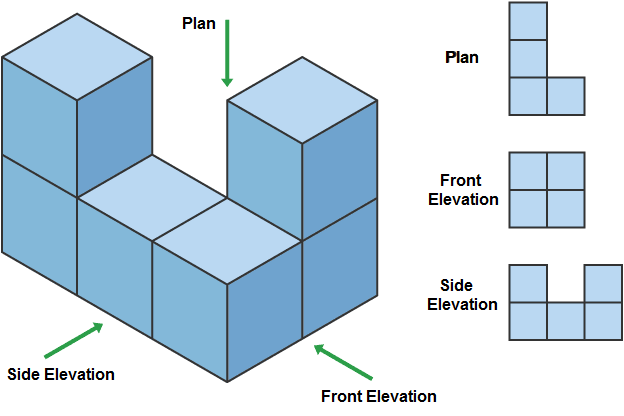## Quantities Associated with a Solid

Faces, edges and vertices

• The corners of a solid shape are called its vertices.
• The line segment joining two vertices is called an edge, or when two planes of a solid meet it forms an edge.
• The surfaces of a solid shape are called as its face.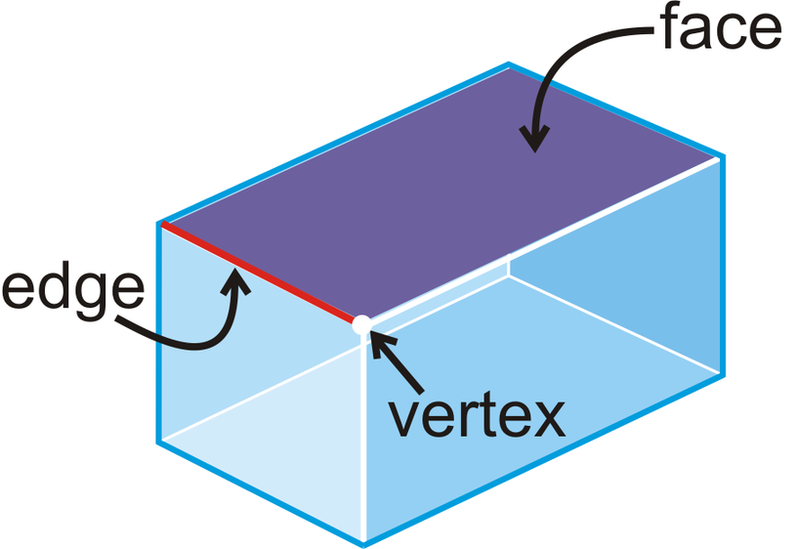The table below shows the number of faces, edges and vertices some solid shapes have.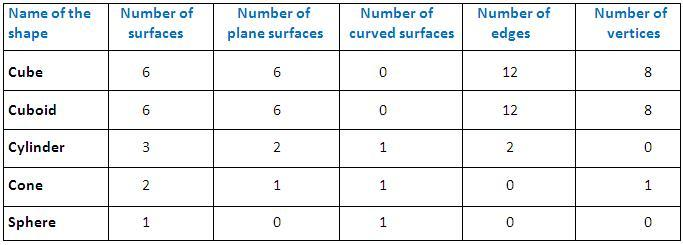## Nets of Solid Shapes

Building 3-D objects

A net is a flattened out skeletal outline or a blueprint of a solid which can be folded along the edges to create solid.The same solid can have multiple nets.

Example: A net for a cube box.To know more about 3D Shapes, visit here.

#### To Know About Nets Of Solid Shapes, Watch The Below Video: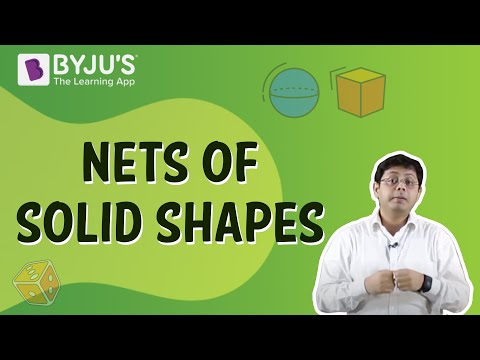## Representing 3D Shapes on a Paper

Drawing solids on a flat surface

• Solid shapes can be represented on a 2D flat surface like paper.
• This is done by drawing oblique sketches, to make it appear 3D. This is called as 2D representation of a 3D solid.

Obliques sketches

• Oblique sketches do not have the exact length of a solid shape but appears exactly like the solid shape.
• Example: Drawing an oblique sketch of a cube:
Step 1: Draw the front and the opposite faces.
Step 2: Join the corresponding corners. (Figure 1)
Step 3: Redraw using dotted lines for hidden edges. (Figure 2)

This gives the oblique sketch of the cube.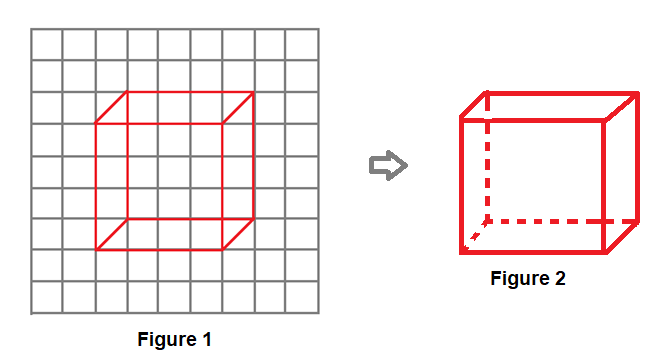Isometric sketches

• Isometric sketches have measurements equal to that of the solid.
• They are usually drawn on an isometric dotted paper.
• Example: Drawing an isometric sketch of a cuboid of dimensions 4 × 3 × 3:

Step 1: Draw a rectangle to show the front face of the cuboid.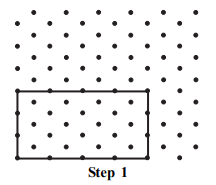Step 2: Draw four parallel line segments of length three starting from the four corners of the rectangle.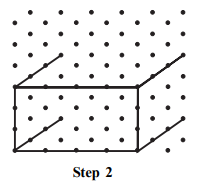Step 3: Connect the corresponding corners using appropriate line segments.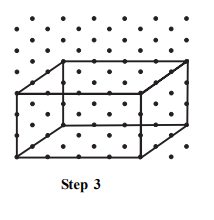Step 4: The figure thus obtained is the isometric sketch of the cuboid.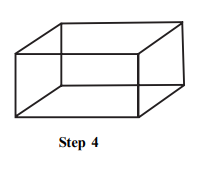#### For More Information On Three Dimensional Shapes, Watch The Below Video.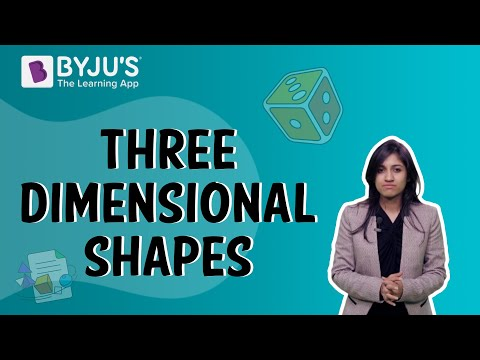## Frequently asked Questions on CBSE Class 7 Maths Notes Chapter 15: Visualising Solid Shapes

### What are the uses of ‘3D objects’?

3D models are used to portray real-world and conceptual visuals for art, entertainment, simulation and drafting.

### What is a ‘Cross section’?

A cross section is the non-empty intersection of a solid body in three-dimensional space with a plane, or the analog in higher-dimensional spaces.

### What is an ‘Isometric sketch’?

Isometric Sketch is a pictorial representation of an object in which all three dimensions are drawn at full scale.

To know more about 3D Shapes, visit here.